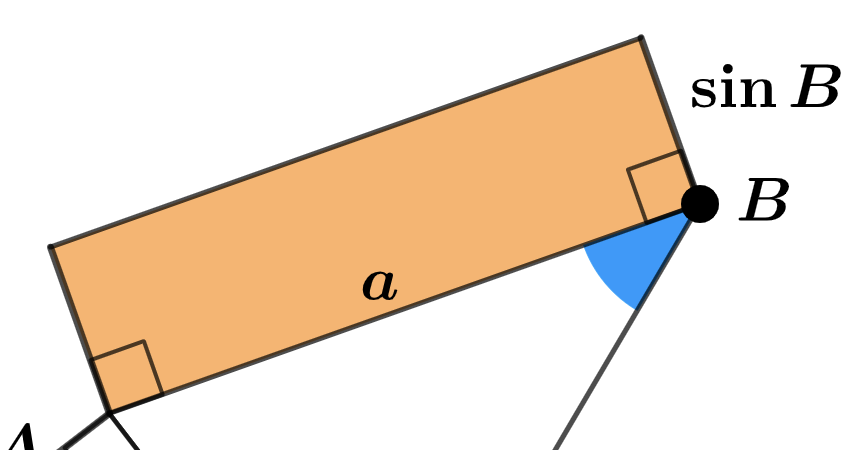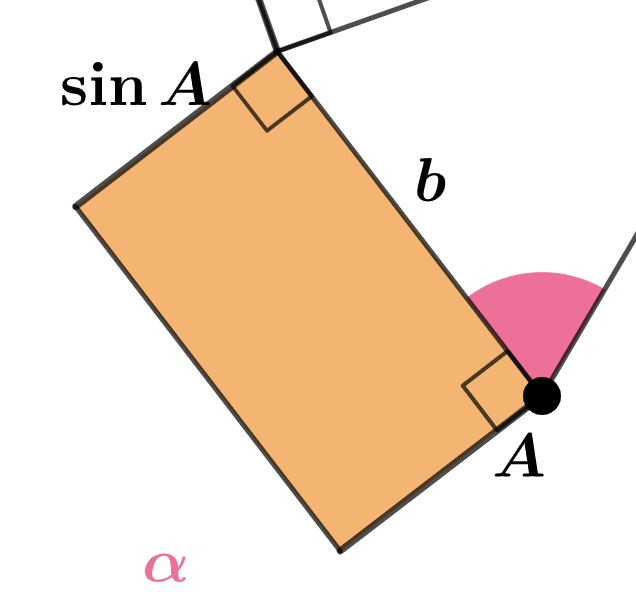# Law of Sines: Intro via Areas

## In the app below, points A and B are MOVEABLE. You can change the sizes of the colored angles using the two colored sliders in the lower left corner. Slide the long slider slowly and carefully watch what happens!What is the area of this rectangle in terms of a and sin B?What is the area of this rectangle in terms of b and sin A?

What can we conclude about the areas of these two rectangles? Why can we conclude this?

Given your responses to the questions above, write an equation that expresses the relationship among a, b, sin A, and sin B.